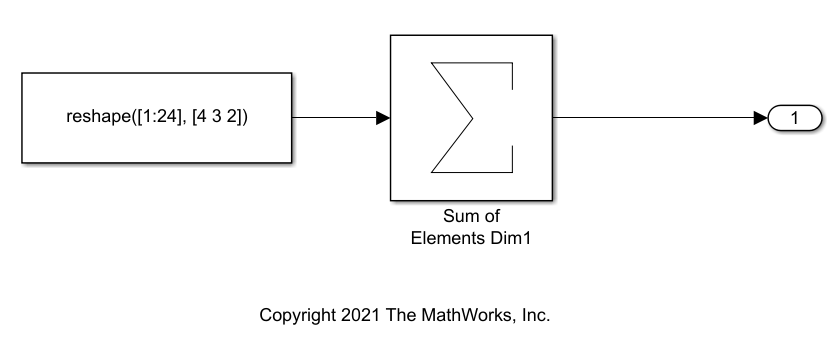# Optimize Code for Reduction Operations by Using SIMD

This example shows how to generate optimized single instruction, multiple data (SIMD) code that performs reduction operations. A reduction is an operation that reduces a set of elements, such as an array, to a single value using an associative binary operator. For example, calculating the sum of the elements in an array is a reduction operation that uses the addition operator. For processors that support SIMD instructions, you can optimize reduction operations by generating parallel code that uses the SIMD instructions for the operation.

### Example Model

Open the example model `ex_rowmajor_algorithm`. The model calculates the sum of the elements in a 4 by 3 by 2 array.

```model = 'ex_rowmajor_algorithm'; open_system(model)```### Generate SIMD Code Without Reduction Optimization

Open the configuration parameters for the model. On the Modeling tab, click Settings. On the Code Generation pane, set System target file to `grt.tlc`. On the Optimization pane, set the Leverage target hardware instruction set extensions to `SSE2`. Alternatively, use the command-line.

```set_param(model, 'SystemTargetFile', 'grt.tlc'); set_param(model, 'SaveOutput', 'off'); set_param(model, 'RTWVerbose', 'off'); set_param(model, 'InstructionSetExtensions', 'SSE2');```

De-select the parameter Optimize reductions.

`set_param(model, 'OptimizeReductions', 'off');`

Build the model.

`slbuild(model);`
```### Starting build procedure for: ex_rowmajor_algorithm ### Successful completion of build procedure for: ex_rowmajor_algorithm Build Summary Top model targets built: Model Action Rebuild Reason ====================================================================================================== ex_rowmajor_algorithm Code generated and compiled Code generation information file does not exist. 1 of 1 models built (0 models already up to date) Build duration: 0h 0m 16.273s ```

Inspect the generated `rtwdemo_simd_reductions_step` function.

```file = fullfile('ex_rowmajor_algorithm_grt_rtw','ex_rowmajor_algorithm.c'); rtwdemodbtype(file,'/* Model step function */','/* End of Sum',1,1);```
```/* Model step function */ void ex_rowmajor_algorithm_step(void) { int32_T i; /* Sum: '<Root>/Sum of Elements Dim1' incorporates: * Constant: '<Root>/Constant1' */ ex_rowmajor_algorithm_Y.Out2 = -0.0F; for (i = 0; i < 24; i++) { ex_rowmajor_algorithm_Y.Out2 += ex_rowmajor_algorithm_ConstP.Constant1_Value[i]; } ```

The generated code contains a non-vectorized loop that adds each array element individually.

### Generate SIMD Code With Reduction Optimization

Open the configuration parameters for the model. On the Modeling tab, click Settings. On the Optimization pane, set the Leverage target hardware instruction set extensions to `SSE2`. Alternatively, use the command-line.

`set_param(model, 'InstructionSetExtensions', 'SSE2');`

Select the parameter Optimize reductions. If you want to optimize reduction operations that operate on floating-point values, set Support: Non-finite numbers to `Off`. Reduction optimization does not support operations on floating-point values when non-finite numbers are supported.

`set_param(model, 'OptimizeReductions', 'on');`

Build the model.

`slbuild(model);`
```### Starting build procedure for: ex_rowmajor_algorithm ### Successful completion of build procedure for: ex_rowmajor_algorithm Build Summary Top model targets built: Model Action Rebuild Reason ===================================================================================== ex_rowmajor_algorithm Code generated and compiled Generated code was out of date. 1 of 1 models built (0 models already up to date) Build duration: 0h 0m 12.186s ```

Inspect the generated `rtwdemo_simd_reductions_step` function.

```file = fullfile('ex_rowmajor_algorithm_grt_rtw','ex_rowmajor_algorithm.c'); rtwdemodbtype(file,'/* Model step function */','/* Matfile',1,1);```
```/* Model step function */ void ex_rowmajor_algorithm_step(void) { int32_T i; /* Sum: '<Root>/Sum of Elements Dim1' incorporates: * Constant: '<Root>/Constant1' */ ex_rowmajor_algorithm_Y.Out2 = -0.0F; for (i = 0; i < 24; i++) { ex_rowmajor_algorithm_Y.Out2 += ex_rowmajor_algorithm_ConstP.Constant1_Value[i]; } /* End of Sum: '<Root>/Sum of Elements Dim1' */ ```

The vectorized loop uses the SSE2 function `mm_add_ps`, which adds four elements of the array to the sum in one step. This optimization improves the execution speed of the generated code.

Open the code generation report. On the C Code tab, click Open Report.On the report summary, in the Additional Information section, the report indicates that reduction operations are optimized using the SSE2 instruction set.